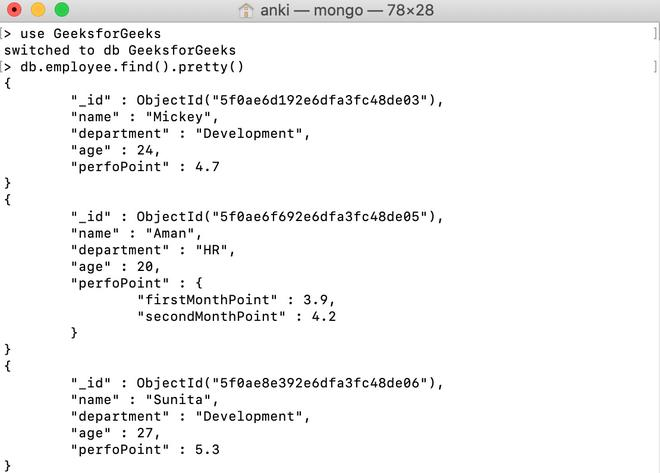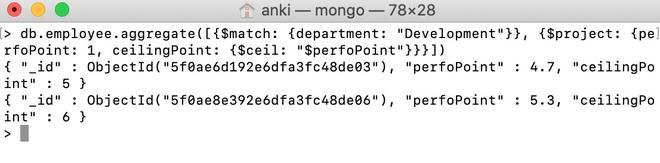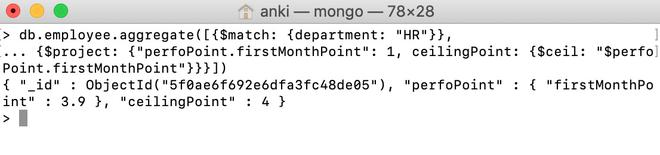GFG App
Open AppBrowser
Continue

MongoDB provides different types of arithmetic expression operators that are used in the aggregation pipeline stages and \$ceil operator is one of them. This operator is used to find the smallest integer greater than or equal to the specified number.

```{ \$ceil: <number> }
```

Here, the number is a valid expression until it resolves to a number.

• If the entered value is null, then this operator will return null.
• If the entered value is NaN, then this operator will return NaN.
• If the entered value is a missing field, then this operator will return null.

Examples:

In the following examples, we are working with:

Database: GeeksforGeeks

Collection: employee

Document: three documents that contain the details of the employees in the form of field-value pairs.Using \$ceil Operator:

In this example, we are going to find the smallest integer greater than or equal to the value of the perfoPoint field in the development department.

```db.employee.aggregate([{\$match: {department: "Development"}},
{\$project: {perfoPoint: 1, ceilingPoint: {\$ceil: "\$perfoPoint"}}}])

```Using \$ceil Operator in the Embedded Document:

In this example, we are going to find the smallest integer greater than or equal to the value of the perfoPoint.firstMonthPoint field in the HR department.

```db.employee.aggregate([{\$match: {department: "HR"}},
... {\$project: {"perfoPoint.firstMonthPoint": 1,
ceilingPoint: {\$ceil: "\$perfoPoint.firstMonthPoint"}}}])

```My Personal Notes arrow_drop_up